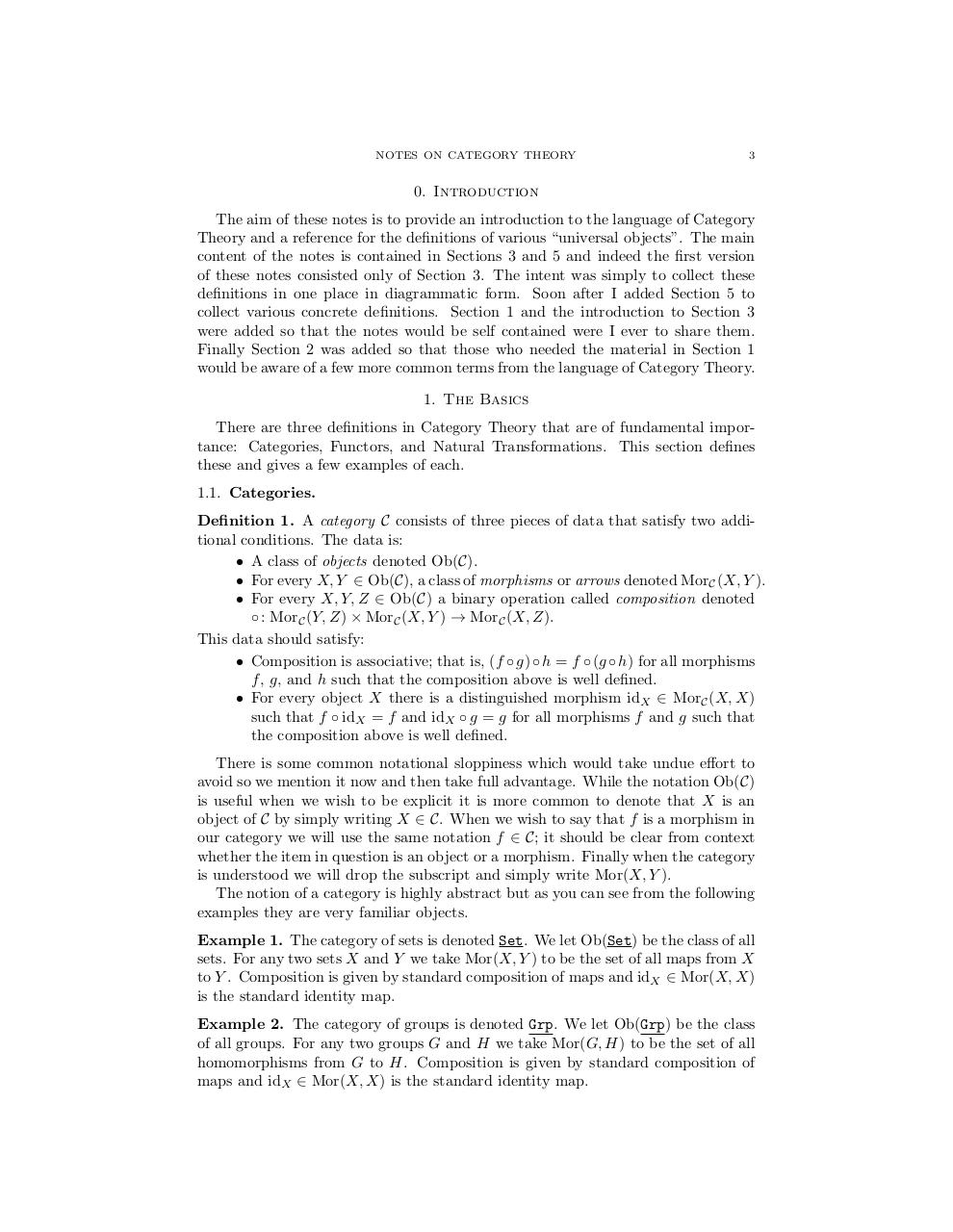# CategoryTheory.pdfPage 1 2 34519

#### Text preview

NOTES ON CATEGORY THEORY

3

0. Introduction
The aim of these notes is to provide an introduction to the language of Category
Theory and a reference for the definitions of various “universal objects”. The main
content of the notes is contained in Sections 3 and 5 and indeed the first version
of these notes consisted only of Section 3. The intent was simply to collect these
definitions in one place in diagrammatic form. Soon after I added Section 5 to
collect various concrete definitions. Section 1 and the introduction to Section 3
were added so that the notes would be self contained were I ever to share them.
Finally Section 2 was added so that those who needed the material in Section 1
would be aware of a few more common terms from the language of Category Theory.
1. The Basics
There are three definitions in Category Theory that are of fundamental importance: Categories, Functors, and Natural Transformations. This section defines
these and gives a few examples of each.
1.1. Categories.
Definition 1. A category C consists of three pieces of data that satisfy two additional conditions. The data is:
• A class of objects denoted Ob(C).
• For every X, Y ∈ Ob(C), a class of morphisms or arrows denoted MorC (X, Y ).
• For every X, Y, Z ∈ Ob(C) a binary operation called composition denoted
◦ : MorC (Y, Z) × MorC (X, Y ) → MorC (X, Z).
This data should satisfy:
• Composition is associative; that is, (f ◦ g) ◦ h = f ◦ (g ◦ h) for all morphisms
f , g, and h such that the composition above is well defined.
• For every object X there is a distinguished morphism idX ∈ MorC (X, X)
such that f ◦ idX = f and idX ◦ g = g for all morphisms f and g such that
the composition above is well defined.
There is some common notational sloppiness which would take undue effort to
avoid so we mention it now and then take full advantage. While the notation Ob(C)
is useful when we wish to be explicit it is more common to denote that X is an
object of C by simply writing X ∈ C. When we wish to say that f is a morphism in
our category we will use the same notation f ∈ C; it should be clear from context
whether the item in question is an object or a morphism. Finally when the category
is understood we will drop the subscript and simply write Mor(X, Y ).
The notion of a category is highly abstract but as you can see from the following
examples they are very familiar objects.
Example 1. The category of sets is denoted Set. We let Ob(Set) be the class of all
sets. For any two sets X and Y we take Mor(X, Y ) to be the set of all maps from X
to Y . Composition is given by standard composition of maps and idX ∈ Mor(X, X)
is the standard identity map.
Example 2. The category of groups is denoted Grp. We let Ob(Grp) be the class
of all groups. For any two groups G and H we take Mor(G, H) to be the set of all
homomorphisms from G to H. Composition is given by standard composition of
maps and idX ∈ Mor(X, X) is the standard identity map.